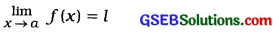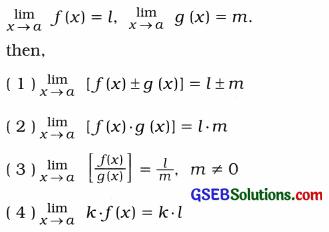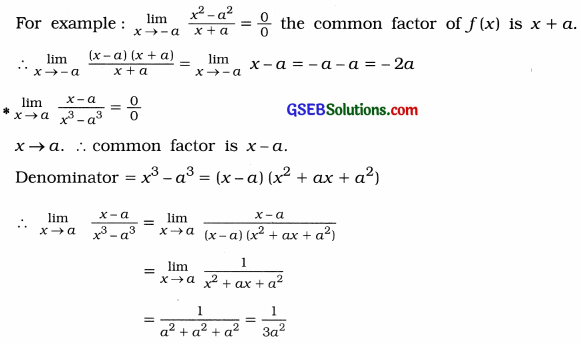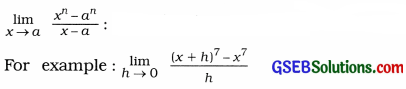# GSEB Class 12 Statistics Notes Part 2 Chapter 4 Limit

This GSEB Class 12 Commerce Statistics Notes Part 2 Chapter 4 Limit Posting covers all the important topics and concepts as mentioned in the chapter.

## Limit Class 12 GSEB Notes

Real Line and its Interval:
Real Line:
A line where its points are the real numbers, is called the real line.

Interval:
A set of reed numbers between any two real numbers is an interval.
1. Closed Interval: If a, be R and a < b, then the set of all real numbers between a and b including a and b is called a closed interval. It is denoted by [a, b]. Thus, [a, b] = (x|a ≤ x ≤ b, x ∈ R}.

2. Open Interval: If a, be R and a < b, then the set of all real numbers between a and b not including a and b is called an open interval. It is denoted by (a, b).
Thus, (a, b) = {x | a < x < b, x ∈ R}.

3. Closed-Open Interval: If a, b ∈ R and a < b, then the set of all real numbers between a and b including a but not including b is called a closed-open interval. It is denoted by la, b).
Thus, [a, b) = {x | a ≤ x < b, x ∈ R}.

4. Open-Closed Interval: If a, be R and a < b, then the set of all real numbers between a and b not including a but including b is called an open-closed interval. It is denoted by (a, b].
Thus, (a, b] = {x | a < x ≤ b, x ∈ R}. Modulus: If a real number is x, then modulus is used to express x as non-negative. The modulus of x is denoted by |x|. The modulus of real number is always positive.
|x| = x, if x > 0
= -x, if x < 0

Meaning of |x – a | < δ:
|x – a | < δ = (x – a) < δ if x ≥ a
or = x < (a + δ) if x ≥ a
= (a – x) < δ if x < a
or = x > (a – δ) if x < a
∴ |x – a| < δ ⇔ x ∈ (a- δ, a + δ)Neighbourhood:
Any open interval containing a, a ∈ R is called a neighbourhood of a.

δ neighbourhood a:
If a ∈ R and δ is non-negative real number, then the open interval (a – δ, a + δ) is called δ neighbourhood of a. It is denoted by N (a, 5).
Thus, N (a, δ) = {x | (a – δ) < x < (a + δ), x ∈ R}
= {x| |x – a| < δ, x ∈ R}
In modulus form : N (a, 5) = |x – a| < δ
In interval form : N (a, 5) = (a – δ, a + δ)

Punctured δ neighbourhood of a:
If a ∈ R and δ is a non-negative real number, then the open interval (a – δ, a + δ)- {a} is called punctured δ neighbourhood of a. It is denoted by N* (a, δ). Thus,
N* (a, 8) = N (a, δ) – {a}
= {x | a – δ < x < a + δ; x ≠ a, x ∈ R}
= {x| |x – a| < δ; x ≠ a, x ∈ R}

Limit of a Function:

• Meaning of x → a: When the value of a variable x is brought very close to a number ‘a’ by increasing or decreasing its value, then it can be said that x tends to a. It is denoted by x → a.
• x → a means that value of x is very close to a but x ≠ a.
• Meaning of x → 0: If by decreasing the positive value of a variable x or by increasing the negative value of variable x, the value x is brought very close to ‘O’, then it can be said that x tends to ‘0’. It is denoted by x → 0.
• x → 0 means that the value of x is very close to ‘0’ but x ≠ 0.

Limit of a Function:
When the value of a variable x is brought closer and closer to a number ‘a’, the value of function fix) approaches closer and closer to a definite number T, then we can say that as x tends to ‘a’ f(x) tends to T that is x a,f (x) —> l. This definite number T is called limit of a function f(x). Symbolically it can be written as follows :l is the limit of f(x). Hence, f(x) ≠ Z.

Important Formulae:
1. Working Rules of Limit:
If f(x) and g(x) are two functions of real variable x and2. Standard Forms of Limit:
(1) If f(x) = a0 + a1x + a0x2 + … + anxn, then3. δ neighbourhood of a :
N (a, δ) = (x| (a – δ) < x < (a + δ), x ∈ R}

4. Punctured 8 neighbourhood of a:

• N * (a, δ) = {x | (a – δ) < x < (a + δ), x ≠ a, x∈ R}
• N (a, δ) = |x – a|< δ (Modulus form)
• N (a, δ) = (a – δ, a + δ) (Interval form)Remember to Find Limit of a Function
1. To find limit by Tabular Method :

 Limiting Value of x Increasing Values Decreasing Values -2 -2.1. -2.01, -2.001, -2.0001,… – 1.9, – 1.99, -1.999, – 1.9999,… – 1 -1.1, -1.01, -1.001, -1.0001,… -0.9, -0.99, -0.999, -0.9999,… 0 -0.1, -0.01, -0.001, -0.0001…. 0.1, 0.01, 0.001, 0.0001,… 1 0.9, 0.99, 0.999, 0.9999,… 1.1, 1.01, 1.001, 1.0001,… 2 1.9, 1.99, 1.999, 1.9999,… 2.1, 2.01, 2.001, 2.0001,…

2. To find limit by putting limiting value of x in function:
1. By putting the limiting value of x In the function f(x), if we get the numerator of f(x) as ‘0’, then the limit of function f(x) is ‘0’.2. By putting the limiting value of x In the function f(x), if we get the denominator of f(x) as ‘0’. then the limit of function f(x) does not exist.3. By putting the limiting value of x In the function f(x). if we get both the numerator and denominator of f(x) as 0 that Is we get $$\frac{0}{0}$$ which is indeterminate. So we cannot find the value of limit of function f(x).In such cases. we factorise numerator and denominator of function and find the limit of function after eliminating the common factor of function.

• If x → a. the common factor of the function will be (x – a).
• If x → – a, the common factor of the function will be (x + a).4. Transforming the function to standard form of limitFor transforming to standard form put x + h = t. So, when h → 0, t → x and h = t — x.